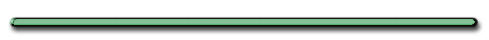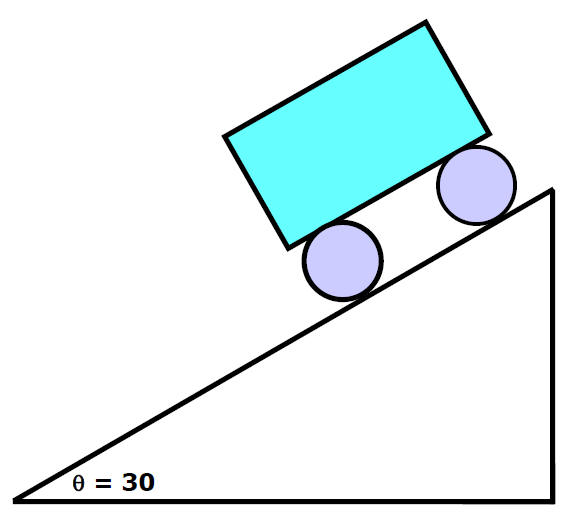HomeMass M, cord with a tension Tf , ramp of angle q.  What is Tf in terms of M, qTf = MgsinqCar at rest, rolls down a 30 degree ramp. How much time to roll 100. m down ramp?d = 100 m Vi = 0 t = ? What else to I need to solve?     a = gsinӨVi = 0 a = gsinq = 9.8 m/s2sin30 t = ? d = 100 m   d = d0 + Vit + 1/2 at2 100. m = 0 + 1/2(4.9 m/s2)t2   t = 6.4 secondsHanging Box©Tony Mangiacapre., - All Rights Reserved [Home] Established 1995 Use any material on this site (w/ attribution)